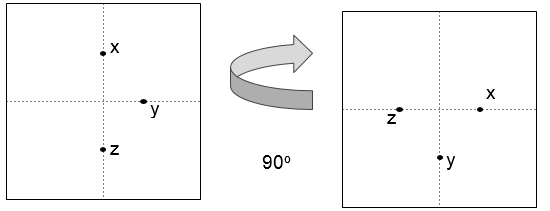# Find if it's possible to rotate the page by an angle or not in C++

In this problem, we are given coordinates of three points that lie on a page. Our task is to find if it’s possible to rotate the page by an angle or not.

The rotation of the page is made in such a way that the new position of ‘x’ is the old position of ‘y’, the new position of ‘y’ is the old position of ‘z’. And print “Yes” or “No” based on the rotation.

## Let’s take an example to understand the problem,

Input: x = (0, 1), y = (1, 0), z = (0, -1)

Output: Yes

Explanation:We can rotate the page by 90o.

Solution Approach:

We can rotate the page by some angle if some conditions are possible.

This can be done if the distance between x and y is the same as the distance between y and z. Also, if all the points lie on the same line rotation cannot be possible.

## Example

Live Demo

#include<bits/stdc++.h>
using namespace std;

int possibleOrNot(int coordinates){

long long dis1 = pow(coordinates - coordinates, 2) + pow(coordinates - coordinates, 2);
long long dis2 = pow(coordinates - coordinates, 2) + pow(coordinates - coordinates, 2);

if(dis1 != dis2)
return 0;
else if (coordinates == ((coordinates + coordinates) / 2.0) &amp;&amp; coordinates == ((coordinates + coordinates) / 2.0))
return 0;
else
return 1;
}

int main() {

int coordinates = {{0 , 1}, {1 , 0}, {0, -1} } ;
if ( possibleOrNot(coordinates))
cout<<"The rotation of page is possible";
else
cout<<"The rotation of page is not possible";

return 0;
}

## Output

The rotation of page is possible